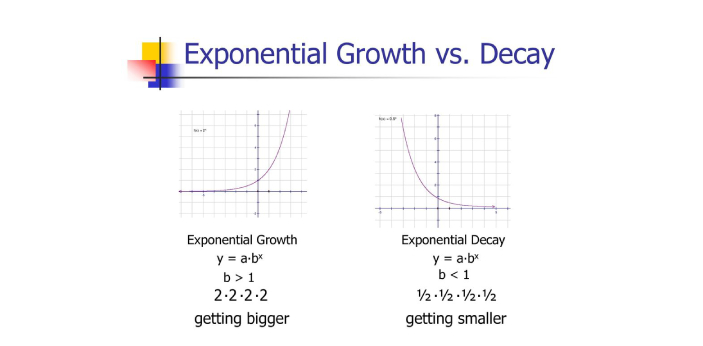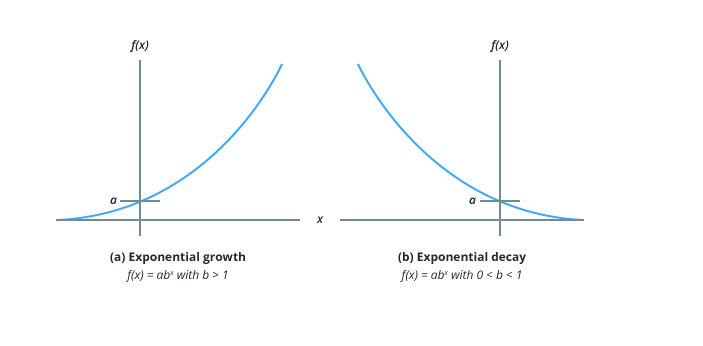What is the difference between Exponential Growth and Exponential - ProProfs Discuss+ Ask Question

# What is the difference between Exponential Growth and Exponential Decay?

Asked by R. Barnes, Last updated: Jun 26, 2022

###Request 0FollowShareAnswer AnonymouslyAnswer LaterCopy LinkM. Porter, Senior Executive, Master of Art, San JoseAn exponential function is any pattern of values that is multiplied continuously by a specific number. Exponential growth occurs when a quantity increases by the same proportion at every given period of time. In mathematical function, the numbers multiply as time goes by.On a graph, this is represented by a curved line that goes upward. Exponential decay occurs when a quantity decreases by the same proportion at every given period of time. On a graph, decay is represented by a curved line that goes downwards. I hope you find this information helpful.Bergeront Tiffney, Computer Engineer, M. Tech, Southeast Montgomery

Exponential growth is used when the base of the exponential is known to be larger than 1. This will make the numbers that are already listed there to become more prominent. It can be considered an exponential decay when people know that the exponential is between 1 and 0. This means that there is a massive tendency for the numbers to become smaller over time.You can tell if something is going through exponential growth when the growth rate is getting higher, and the numbers keep on improving. At times when the growth rate may not be too good, this may signify that they are experiencing exponential decay.Search for Google imagesSelect a recommended image
Upload from your computerCancelSearch for Google imagesSelect a recommended image
Upload from your computerCancelSearch for Google imagesSelect a recommended image
Upload from your computerCancel